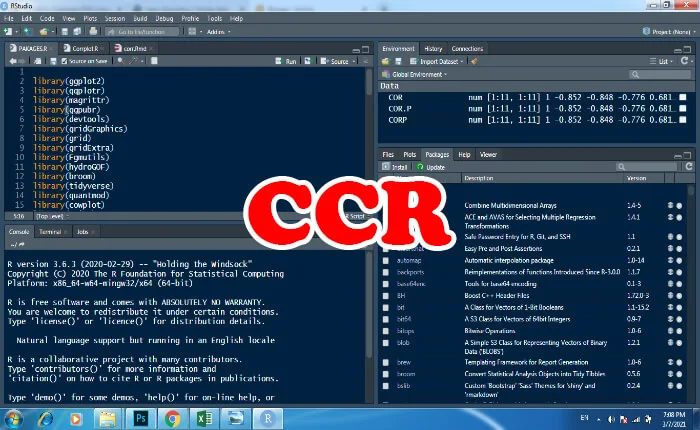# Calculate correlation coefficient r using rstudio

## Calculate Correlation Coefficient r using RStudio### Correlation coefficient r

In statistics, the correlation coefficient r is a statistical measure of the strength and direction of a linear relationship between two variables. The Correlation coefficients are expressed as values between +1 and -1. So, how to interpret the correlation coefficient r. r = 1: indicates a strong positive relationship between the variables, r = -1: indicates a strong negative relationship between the variables and r = 0: indicates no relationship between the variables.

A correlation is the relationship between two variables: variable observed (Obs: observed values) and variable simulated (Sim: simulated values). In this lesson we will calculate three correlation coefficients of: Pearson, Kendall and Spearman. All this coefficient measures the strength of the relationship between the variables.

Pearson’s Correlation Coefficient. It is known as the best method of measuring the relationship between variables (observed and simulated). It gives information about the magnitude of the correlation, as well as the direction of the relationship.

### Application

In this example we will use the data of three climate stations. you can add other stations in the script.
Cor(Obs,Sim, method ="pearson") Default method is pearson.
Cor(Obs,Sim, method ="kendall"), Cor(Obs,Sim, method ="spearman")
Obs : observed values
Sim : simulated values

Examples
cor(data_source\$observed , data_source\$simulated ,method ="pearson")
cor(data_source\$observed , data_source\$simulated ,method ="kendall")
cor(data_source\$observed , data_source\$simulated ,method ="spearman")

Create and save your R Script
Pearson
cor.s1p<- cor(data_source\$observed ,data_source\$simulated , method="pearson")
cor.s1p
Kendall
cor.s1k<- cor(data_source\$observed ,data_source\$simulated , method="kendall")
cor.s1k
Spearman
cor.s1s<- cor(data_source\$observed ,data_source\$simulated , method="spearman")
cor.s1s
Calculate Correlation Coefficient r using R Studio